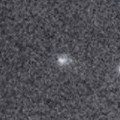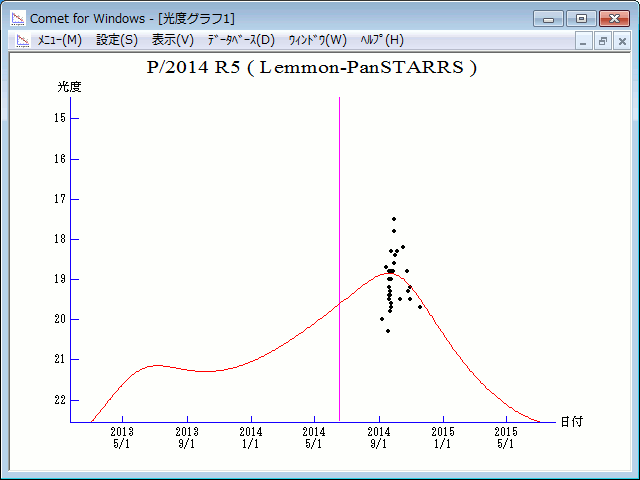# \$B%l%b%s(B-\$B%Q%s%9%?!<%:WB@1(B

445P/Lemmon-PanSTARRS (2014)###\$B%W%m%U%#!<%k(B

 \$BId9f(B 445P/2014 R5 \$BH/8+F|(B 2014\$BG/(B9\$B7n(B14\$BF|!"(B19\$BF|(B \$BH/8+8wEY(B 19.1\$BEy!"(B20.5\$BEy(B \$BH/8+ Mt. Lemmon Survey, Pan-STARRS 1 telescope (Haleakala)

###\$B###\$B50F;MWAG(B

```                    Epoch = 2014 July  2.0 TT
T = 2014 June 17.58594 TT        Peri. = 213.87614
e = 0.4127512                    Node  = 126.54501 2000.0
q = 2.3863022 AU                 Incl. =   1.08823
a =  4.0635286 AU   n = 0.12032312   P =   8.19 years
```

###\$B8wEYJQ2=(B

```        m1 = 14.0 + 5 log\$B&\$(B + 10.0 log r
```##### \$B50F;MWAG\$O!"(BCBET 5149\$B\$K7G:\\$5\$l\$?\$b\$N\$G\$9!#(B \$B8wEY%0%i%U\$O(BComet for Windows\$B\$G:n@.\$7\$?\$b\$N\$G\$9!#(B